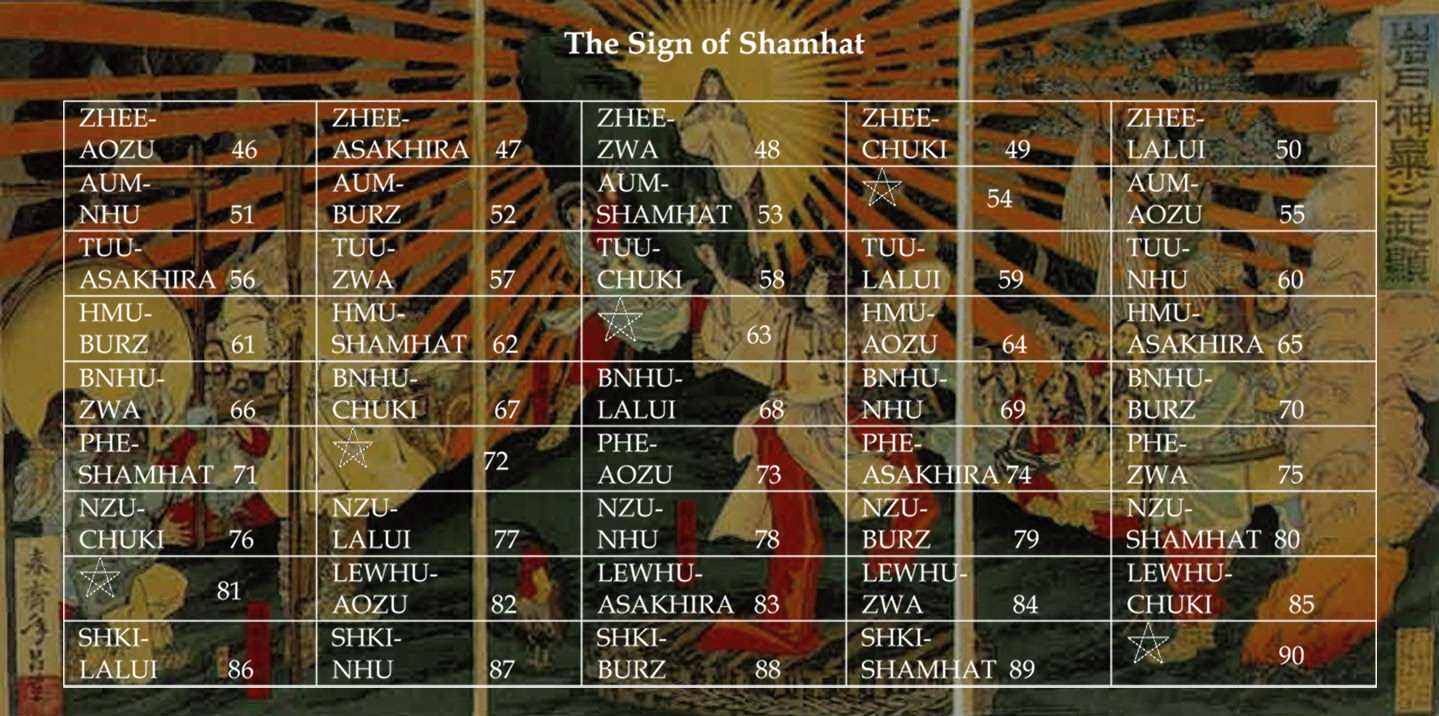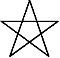Art, History, Music, Politics, and Spirituality For The Modern Alchemist – circulation in over 129 countries

# The Sign of Shamhat Year 18,004: Begins May 3rd 2016Sign of Shamhat Year 18,003 Begins May 3rd 2016

There are eight months in the Calendar of Mu, sometimes referred to as the Nyarzirian Calendar. Each month is consists of forty-five days, and represents a system of the eight hexagrams moving through the five elements. The Ninzuwu Shaman invokes the mudra/mantra formula associated with each hexagram that rules a particular day after invoking the Armor of Amaterasu Ohkami. The chart below illustrates the daily Vasuh letters invoked and the ruling “Ninzuwu” for the said hexagram:
1. May 3rd 2016 = Aozu = 17thHexagram = Phe-Hmu-Tuu
2. May 4th 2016 = Asakhira = 28thHexagram = Aum-Nzu-Bnhu
3. May 5thh2016 = Zwa = 31st Hexagram = Aum-Aum-Hmu
4. May 6th 2016 = Chuki = 43rdHexagram = Zhee-Zhee-Phe
5. May 7th 2016 = Lalui = 45thHexagram = Nzu-Hmu-Lewhu-Tuu-Nzu
6. May 8th 2016 = Nhu = 47thHexagram = Shki-Zhee-Bnhu
7. May 9th 2016 = Butz = 49thHexagram = Hmu-Shki-Aum
8. May 10th 2016 = Shamhat = 58thHexagram = Bnhu-Aum
9. May 11th 2016 =Entering Earth Element
10. May 12th 2016 = Aozu = 17thHexagram = Phe-Hmu-Tuu
11. May 13th 2016 = Asakhira = 28thHexagram = Aum-Nzu-Bnhu
12. May 14th  2016 = Zwa = 31st Hexagram = Aum-Aum-Hmu
13. May 15th t2016 = Chuki = 43rdHexagram = Zhee-Zhee-Phe
14. May 16th 2016 = Lalui = 45thHexagram = Nzu-Hmu-Lewhu-Tuu-Nzu
15. May 17th 2016 = Nhu = 47thHexagram = Shki-Zhee-Bnhu
16. May 18th 2016 = Butz = 49thHexagram = Hmu-Shki-Aum
17. May 19th 2016 = Shamhat = 58thHexagram = Bnhu-Aum
18. May 20th  2016 =Entering Water Element
19. May 21st  2016 = Aozu = 17thHexagram = Phe-Hmu-Tuu
20. May 22nd  2016 = Asakhira = 28thHexagram = Aum-Nzu-Bnhu
21. May 23rd  2016 = Zwa = 31st Hexagram = Aum-Aum-Hmu
22. May 24th  2016 = Chuki = 43rdHexagram = Zhee-Zhee-Phe
23. May 25th  2016 = Lalui = 45thHexagram = Nzu-Hmu-Lewhu-Tuu-Nzu
24. May 26th 2016 = Nhu = 47thHexagram = Shki-Zhee-Bnhu
25. May 27th 2016 = Butz = 49thHexagram = Hmu-Shki-Aum
26. May 28th 2016 = Shamhat = 58thHexagram = Bnhu-Aum
27. May 29th 2016 =Entering Fire Element
28. May 30th 2016 = Aozu = 17thHexagram = Phe-Hmu-Tuu
29. May 31st 2016 = Asakhira = 28thHexagram = Aum-Nzu-Bnhu
30. June 1st 2016 = Zwa = 31st Hexagram = Aum-Aum-Hmu
31. June 2nd 2016 = Chuki = 43rdHexagram = Zhee-Zhee-Phe
32. June 3rd 2016 = Lalui = 45thHexagram = Nzu-Hmu-Lewhu-Tuu-Nzu
33. June 4th 2016 = Nhu = 47thHexagram = Shki-Zhee-Bnhu
34. June 5th 2016 = Butz = 49thHexagram = Hmu-Shki-Aum
35. June 6th h2016 = Shamhat = 58thHexagram = Bnhu-Aum
36. June 7th 2016 =Entering Air Element
37. June 8th 2016 = Aozu = 17thHexagram = Phe-Hmu-Tuu
38. June 9th 2016 = Asakhira = 28thHexagram = Aum-Nzu-Bnhu
39. June 10th 2016 = Zwa = 31st Hexagram = Aum-Aum-Hmu
40. June 11th 2016 = Chuki = 43rdHexagram = Zhee-Zhee-Phe
41. June 12th 2016 = Lalui = 45thHexagram = Nzu-Hmu-Lewhu-Tuu-Nzu
42. June 13th 2016 = Nhu = 47thHexagram = Shki-Zhee-Bnhu
43. June 14th 2016 = Butz = 49thHexagram = Hmu-Shki-Aum
44. June 15th t2016 = Shamhat = 58thHexagram = Bnhu-Aum
45. June 16th 2016 =Entering Void Element

#### You may have missed#### What I Believe by Chris Beland#### One Breath Away by Radio Drive – An Astonishing EP